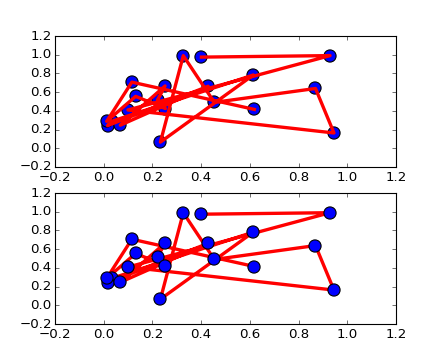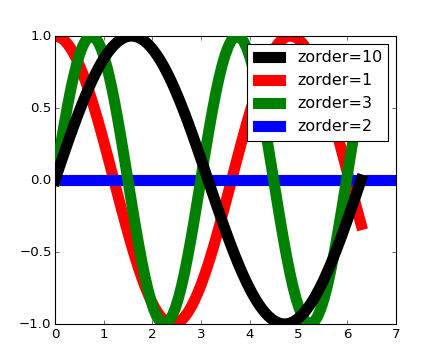### We're updating the default styles for Matplotlib 2.0

Learn what to expect in the new updates#### Previous topic

pylab_examples example code: xcorr_demo.py

#### Next topic

shapes_and_collections Examples

# pylab_examples example code: zorder_demo.py¶(png, hires.png, pdf)(png, hires.png, pdf)

```#!/usr/bin/env python
"""
The default drawing order for axes is patches, lines, text.  This
order is determined by the zorder attribute.  The following defaults
are set

Artist                      Z-order
Patch / PatchCollection      1
Line2D / LineCollection      2
Text                         3

You can change the order for individual artists by setting the zorder.  Any
individual plot() call can set a value for the zorder of that particular item.

In the fist subplot below, the lines are drawn above the patch
collection from the scatter, which is the default.

In the subplot below, the order is reversed.

The second figure shows how to control the zorder of individual lines.
"""

from pylab import *
x = rand(20); y = rand(20)

subplot(211)
plot(x, y, 'r', lw=3)
scatter(x,y,s=120)

subplot(212)
plot(x, y, 'r', zorder=1, lw=3)
scatter(x,y,s=120, zorder=2)

# A new figure, with individually ordered items
x=frange(0,2*pi,npts=100)
figure()
plot(x,sin(x),linewidth=10, color='black',label='zorder=10',zorder = 10)  # on top
plot(x,cos(1.3*x),linewidth=10, color='red', label='zorder=1',zorder = 1) # bottom
plot(x,sin(2.1*x),linewidth=10, color='green', label='zorder=3',zorder = 3)
axhline(0,linewidth=10, color='blue', label='zorder=2',zorder = 2)
l = legend()
l.set_zorder(20) # put the legend on top

show()
```

Keywords: python, matplotlib, pylab, example, codex (see Search examples)